# Drop an Octave: Reducing Spatial Redundancy in Convolutional Neural Networks with Octave Convolution

## 1. 尺度空间理论（scale-space theory）

$L(\cdot,\cdot;t) = g(\cdot,\cdot;t) * f(\cdot,\cdot)$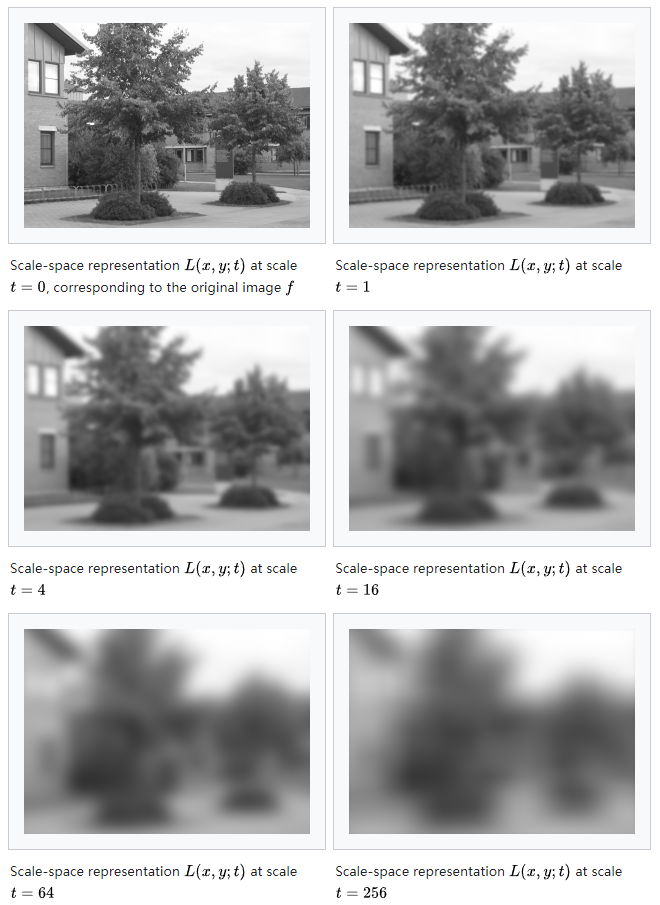## 2. OctConv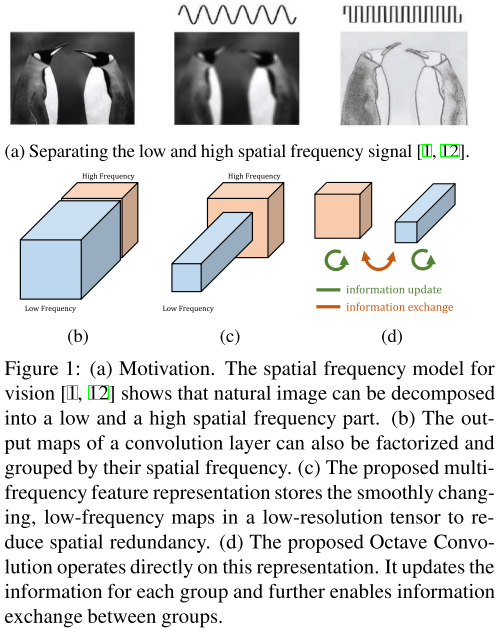$W^H$既有负责$X^H$$Y^H$的部分$W^{H \to H}$也有负责$X^L$$Y^H$的部分：$W^{L \to H}$，即$W^H = [W^{H \to H}, W^{L \to H}]$

$W^L$同理，但对$W^{H \to L}$先执行的是降采样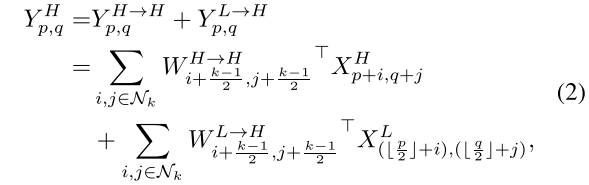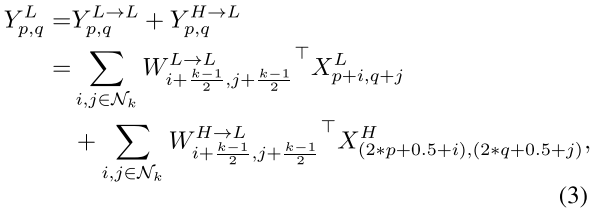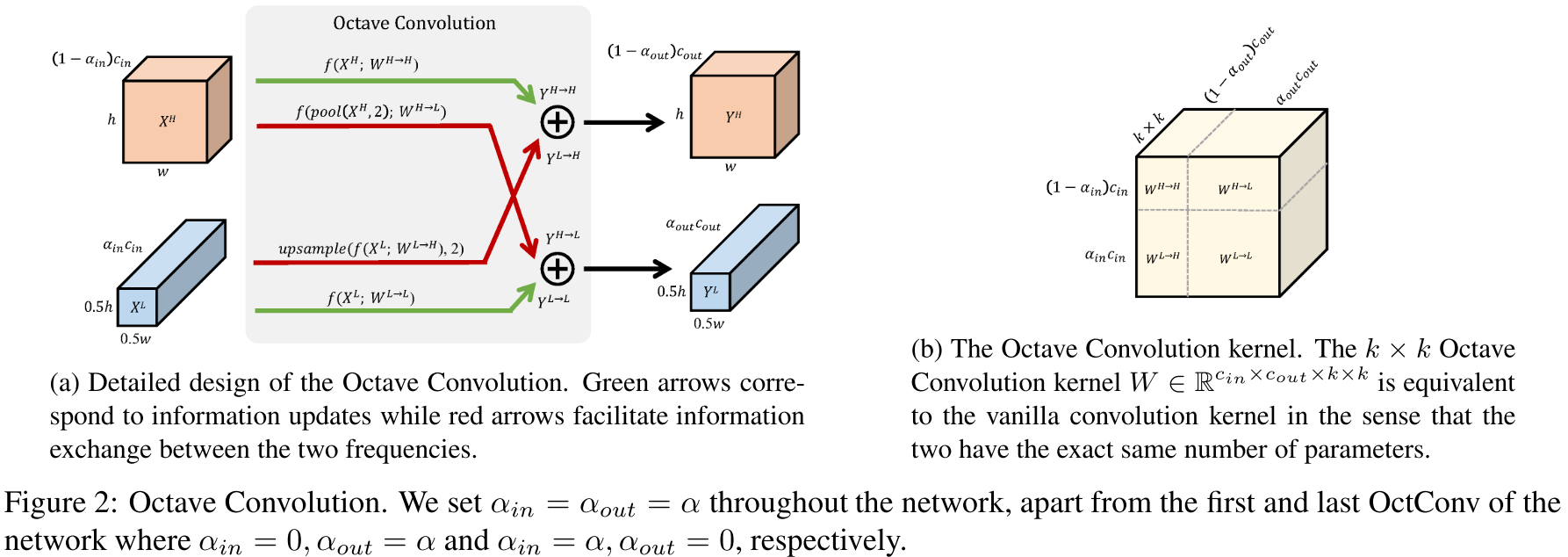## 3. 启发

1. 我们要让神经网络更好地学习。

该文通过尺度空间变换和Octave卷积操作，让网络更清晰地分开处理高、低频分量，并且在低频分量上节约了计算量

又比如BN技巧，也是让网络自我学习$\alpha$$\beta$参数，从而实现特征中心化。

神经网络很厉害，自学能力超强，但我们要适度改造它，让它更快、更精简、更强大。

2. 我们要多从人类视觉特性上思考问题。

因为人类视觉编码的能力远远超过现有技术：分辨率大概是10亿像素（10亿个视杆/视锥细胞），但眼睛到大脑的通路带宽只有8Mbps（1k个神经节）。

本文思考的低频分量冗余，很有可能就是人类视觉编码特性中主要解决的冗余之一。

posted @ 2019-04-16 21:31  RyanXing  阅读(...)  评论(...编辑  收藏